Courses

# Matrix MCQ - 1

## 20 Questions MCQ Test Topic-wise Tests & Solved Examples for IIT JAM Mathematics | Matrix MCQ - 1

Description
This mock test of Matrix MCQ - 1 for Mathematics helps you for every Mathematics entrance exam. This contains 20 Multiple Choice Questions for Mathematics Matrix MCQ - 1 (mcq) to study with solutions a complete question bank. The solved questions answers in this Matrix MCQ - 1 quiz give you a good mix of easy questions and tough questions. Mathematics students definitely take this Matrix MCQ - 1 exercise for a better result in the exam. You can find other Matrix MCQ - 1 extra questions, long questions & short questions for Mathematics on EduRev as well by searching above.
QUESTION: 1

Solution:
QUESTION: 2

Solution:
QUESTION: 3

###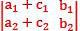can be written as a sum of a determinants

Solution:
QUESTION: 4

If the system of equations x — 2y — 3z = 1, ( P + 2 )z = 3, (2 P + l ) y + z = 2 inconsistent, then value of P is

Solution:
QUESTION: 5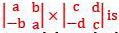Solution:
QUESTION: 6

The value of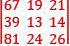is equal to

Solution:
QUESTION: 7

The values of the minors of the elements of the first row of the determinant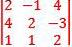are respectively

Solution:
QUESTION: 8

The system of linear equation
x – y + 2z = b1
x + 2y – z = b2
2y – 2z = b
is inconsistent when (b1, b2, b3) equals

Solution:

The system of linear equation
x – y + 2z = b1
x + 2y – z = b2
2y – 2z = b2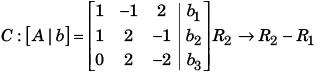Apply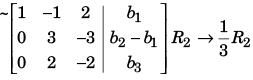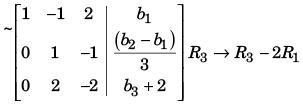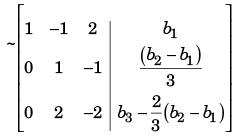System is in consistent if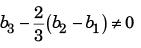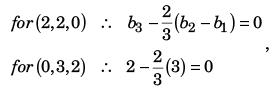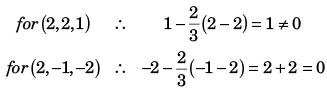QUESTION: 9

The system of equations
2x + 2y – 3z = 1,
4x + 4y + z = 2,
6x + 6y – z = 3 has

Solution:

The matrix form of the system of equation is AX = b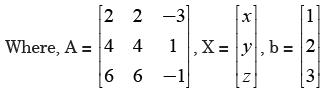The augmented matrix is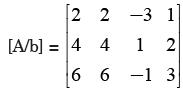Applying R2 → R2 - 2R1 , R3  → R3 - 3R1, we have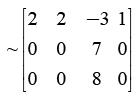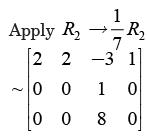Apply R3 → R3 - 8R2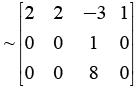Hence, the rank of the matrix is equal to 2 i.e. r ( A | b) = 2.
Here, rank(A) = 2 = rank[A|b] < no of unknowns = 3. So there are infinite many solution of the system of equations.

QUESTION: 10

Square matrix A of order n over R has rank n. Which one of the following statement is not correct?

Solution:

Since, if A is a square matrix of rank n, then it cannot be a singular

QUESTION: 11

The system of equations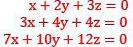Solution:
QUESTION: 12

The given system of equation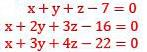Solution:
*Multiple options can be correct
QUESTION: 13

Solution:
*Multiple options can be correct
QUESTION: 14

Which of the following statements is/are correct. The trace of a matrix A =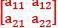is defined by Tr(A) = a11 + a22

Solution:
QUESTION: 15

Which of the following statements is correct?

Solution:

Explanation : Properties of Transpose :

(A + B)T = AT + BT

(kA)T = kAT

*Multiple options can be correct
QUESTION: 16

Which of the following(s) is/are correct?

Solution:
*Multiple options can be correct
QUESTION: 17

Which of the following(s) is/are correct?

Solution:
*Multiple options can be correct
QUESTION: 18

Which of the following(s) is/are correct?

Solution:
*Multiple options can be correct
QUESTION: 19

Which of the following(s) is/are correct?

Solution:
*Multiple options can be correct
QUESTION: 20

Which of the following(s) is/are correct?

Solution: